# Chapter 7 Classic Options

The Classic Options Pricer allows the user to price and analyze the greeks of three products: European, American and Digital Options (Calls and Puts).

## 7.1 European Options

European Options and the behavior of their Greeks have already been largely discussed in chapter 4 and chapter 5 respectively.

The Classic Options Pricer offers a perfect opportunity to put all this theory into practice.

## 7.2 American Options

American options can be exercised at any time during their life. Since investors have the freedom to exercise their American options at any point during the life of the contract, they are logically more valuable than European options.

The Classic Options Pricer prices American Options using the approximation method of Bjerksund and Stensland (1993).

### 7.2.1 American Calls

For American Calls, early exercise may be optimal just before the dividend payment if the dividend payment is large enough.

This can be expressed by the following condition: $$\frac{D}{K} > r * (T-t)$$.

Intuitively, if one exercises the American call, he pays a specific amount of money to buy the underlying shares. On the one hand, he doesn’t receive interest on this cash amount; and, on the other, he would receive future dividends for holding the stocks. In other words, if the dividend yield is higher than the interest rate until maturity, it is optimal to exercise the American call. For stocks not paying dividends, it is never optimal to exercise the American call.

### 7.2.2 American Puts

Ultimately, it can be optimal for the holder of an American put option to choose to exercise if the interest rate that would be received on a cash deposit equal to K is higher than the dividend payments until maturity. For non-dividend-paying stocks, an American put should always be exercised when it is sufficiently deep ITM.

It is important to realize that it makes no sense to exercice an option when there is time premium remaining because you are throwing away that time premium by doing so. You would be better of selling the option than exercising it.

## 7.3 Digital Options

Digital options are quite straigthforward. They are options that pay a fixed coupon if the underlying is below or above a predetermined level and does not give a payout at all in all other cases.

Digitals are still considered as exotic options as they cannot be perfectly replicated by a set of standard options.

### 7.3.1 European Digitals

We will focus on Digital Calls but the same reasoning can always be applied in the case of Digital Puts.

European Digital Calls pay a fixed coupon C if the underlying spot price at maturity T is higher than a predetermined barrier level, K.

The Digital Call payoff can be expressed as: C * $$1_{\{S_T > H\}}$$. This is why we also call them binary Calls.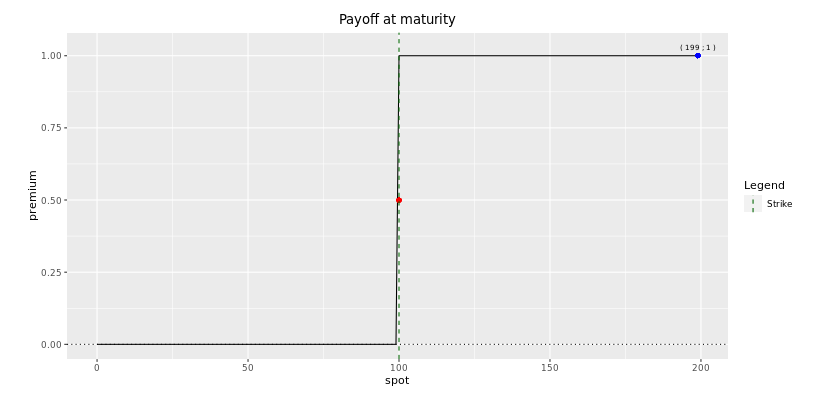Under Black–Scholes, the price of such an option is given by the following formula:

$$\boxed{\text{Digital Call} = C * N(d_2) * e^{-rT}}$$

So the Digital Call price is given by $$N(d_2)$$, which is nothing but the negative of the derivative with respect to K. It gives the probability that the spot at time T is higher than the barrier level.This shape should be familiar to you by now. It looks like the Delta of a European Call. This is due to the fact that this Delta is given by $$N(d_1)$$, which is also a cumulative distribution function.

If the shape of the premium looks like the shape of the delta of European call, then the delta of a digital call will be the gamma of a vanilla call. As we will see, this means that we no longer have this bounded delta from zero to one.

As you will see, Digital Calls are used extremely often in the world of structured products as their payoffs generally contain some sort of discontinuity.

#### 7.3.1.2 Replication of European Digital Options

The digital call can be thought of as a limit of a call spread. One can therefore make a good estimate of the price of a digital option by using option spreads.

Digital (K) = $$\text{lim}_{\epsilon \rightarrow 0} \; \frac{1}{2 \epsilon} \left( Call(K-\epsilon) - Call(K+\epsilon)\right) = - \frac{\partial Call(K)}{\partial K}$$

As the distance between the call option strikes and the digital strikes, $$\epsilon$$, gets smaller, we need $$\frac{1}{\epsilon}$$ call spreads of width $$2\epsilon$$ to replicate the digital. In the limit, meaning as $$\epsilon$$ approaches zero, the call spread replicates the digital exactly.

Note that the above expression is theoretical as, in practice, a trader will not center the call spread around the barrier. He will be more defensive and take a call spread that over-replicates the digital as shown below.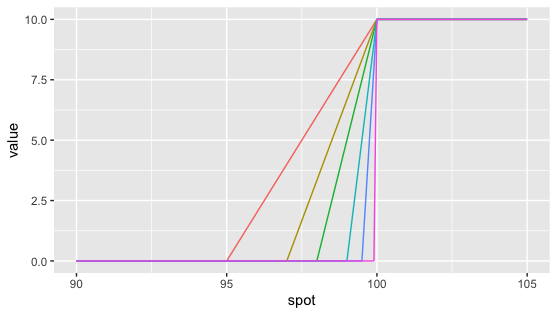#### 7.3.1.3 Hedging a Digital

Well, you should not be surprised if I tell you that the only real way to risk manage the digital option is with option spreads.

You can then hedge a digital call as a call spread. The gearing of the call spread used to over-replicate the digital depends on the strike width of the call spread. The wider the call spread, the lesser the gearing and the more conservative the price.

What do we mean by saying that the call spread over-replicates the digital option?

Let us have a look at Fig 6.3. here above.

Above the barrier level, the call spread has the same payoff as the digital call. Below the barrier level, the digital call has a zero payoff but the call spread has a non-zero payoff between its lower strike and its upper strike located at the barrier level. Therefore, we say that the call spread over-replicates the digital call because its payoff (and therefore its premium) is always greater or equal to the digital call's payoff.

Let us take a small practical example.

As an investor, you buy a 6-month European digitall call on AB Inbev which pays 10€ if after 6 months the ABI stock trades above 50€ and pays 0 if the ABI stock trades below 50€ at maturity.

As a trader, I sell you this digital call on ABI stock. How much will I sell it to you? Well, I will replicate the digital using a geared call spread. I believe that a 2€ wide call spread should be enough for me to risk manage this position. So I will price the digital call as if it was a 48€/50€ call spread that is 5 times geared.

You can think of different scenarii and see that this call spread over-replicates the digital call.

By doing so, I have therefore priced the digital conservatively. I could have been more aggressive by choosing a tighter 49€/50€ call spread. But remember that I have risks to manage, especially gamma and pin risk around the 50€ barrier level.

The smaller the call spread, the more aggressive the price but the more difficult the hedging.

For a digital option, Gamma can be quite large and tricky near the barrier at maturity. Think about the situation where you are just before expiry, the ABI stock trades at 50€ so that the digital would not pay you anything. If ABI stock goes up to 50.02, the digital would suddenly pay you 10€. As a trader, this would be extremely difficult to hedge. As a trader, the call spread gives me a cushion against this risk.

Using a call spread allows to smooth the Greeks. The smaller the call spread, the larger Gamma and Vega can get near the barrier. In fact, around the barrier level, they shoot up and then shoot down while changing sign.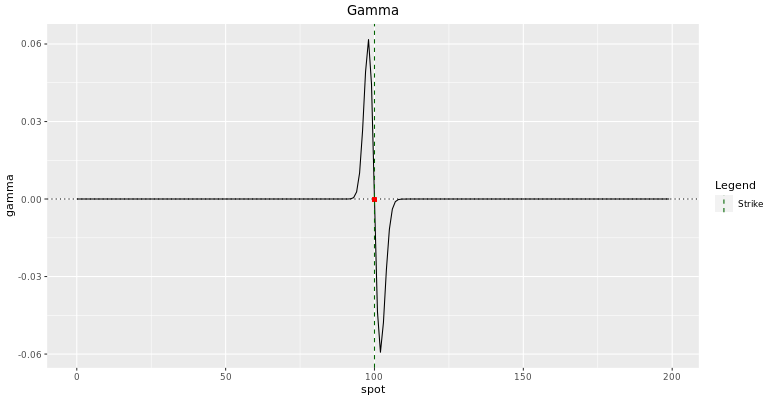We will analyze Call Spreads in more details in the next chapter. You will see that its gamma is smoother than that of a digital call. The larger the strike width, the more this is true.

You shoud have understood by now that when I sell a digital call, I actually book and trade a call spread in my risk management system. As the underlying gets closer to the barrier, you still want to be able to manage your delta hedge properly. A large Gamma means that you will have to buy/sell a large Delta of the underlying, which might be difficult in the market. It is the reason why the liquidity of the underlying is an important variable when selecting the strike width of the replicating call spread.

#### 7.3.1.4 Width of the Call Spread and Barrier Shifts

So the width of the option spread is used as a pricing mechanism to go conservative on the price of a digital option over its model price.

It is necessary in the pricing mechanism to account for real-world difficulty in executing large deltas at the barrier that the model does not consider.

The optimal width of the call spread depends on several parameters among which the size of the digital, the size of the nominal, the underlying's liquidity, the peak delta around the barrier and the implied volatility around the barrier.

In practice, some traders rather take a constant shift of the barrier. Basically, it allows them to take an additional margin for managing the risks if the underlying was to get close to the barrier. This can be more efficient when risk managing a large book of exotic options.

When taking a barrier shift, a trader is pricing a new digital whose replicating centered call spread is the hedge of the actual digital.

The direction of the barrier shift obviously depends on the trader's position.

We will discuss further about barrier shifts in the chapter on barrier options.

#### 7.3.1.5 Risk Analysis - The Greeks

We will shortly speak about the greeks of a digital call at initiation. Note that the risks and therefore the greeks are dynamics. For example, the greeks will be quite different if you get closer to maturity. As I cannot describe every scenario, the best way for you to learn this material is to use the pricer, ask yourself many questions and find your answer using the pricer. For example, what happens to the greeks if just before maturity the spot price is exactly at the barrier level? You open the pricer, you select Digital call in the option type input, you set the stock price at the strike level and you set the maturity close to 0. You will be able to calculate the greeks and see all the related graphics. You will then have to interpret them. If you have any questions, you can always drop us an email at info$@$derivativesacademy.com

##### 7.3.1.5.1 Delta

The holder of a digital call is always long the forward price since a higher forward increases the probability of the option finishing in-the-money.

Being long the forward means being: - Long interest rate - Short dividends - Short borrow costs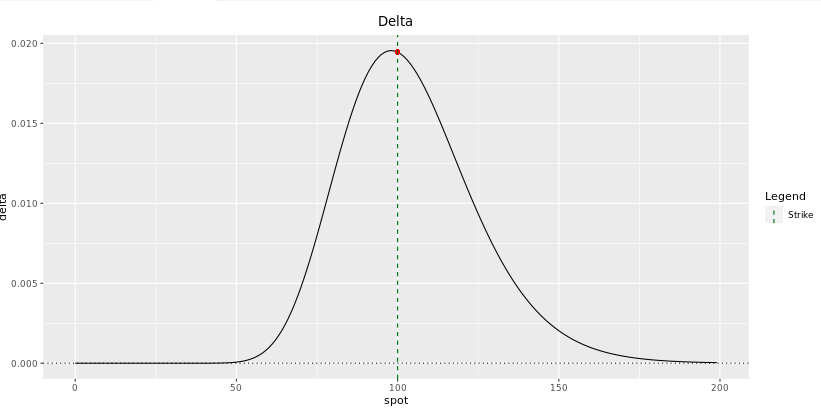Fig: 7.5 :Delta of a 1-year Digital Call at initiation

I don't think I am making you a favor if I describe all the graphics with precision. The best way to develop yourself is to decipher these plots by yourself. For example, you should be able to understand why does the delta converges to zero (and not to 1 as in the case of European calls) when the stock price increases well above the barrier level. Note that the plot of the delta is simply the first derivative of the premium plot with respect to the spot price.

Since a digital call has positive delta, the trader selling it will have to buy delta of the underlying. Therefore the trader will be long dividends, short interest rates and long borrow costs of the underlying.

##### 7.3.1.5.2 Gamma

While their magnitudes are quite different, Gamma and Vega behave similarly and depend about the position of the forward price regarding the barrier. The Gamma plot can be easily deduced from the Delta plot since it is simply the first derivative with respect to the spot price. Unlike vanilla options, the gamma of digital options change sign around the barrier level. While this change is quite smooth at initiation, we have seen that it gets more spiky closer to maturity. It makes the hedging process particularly difficult for the trader as vega and gamma shoot up and down while changing sign.

Think about being the trader hedging this digital call close to maturity when the spot is around the barrier level. How does this change of sign impact your delta hedging?

This discontinuity risk (gap risk) has been discussed and is the reason why barrier shifts are applied and option spreads are used to smooth it.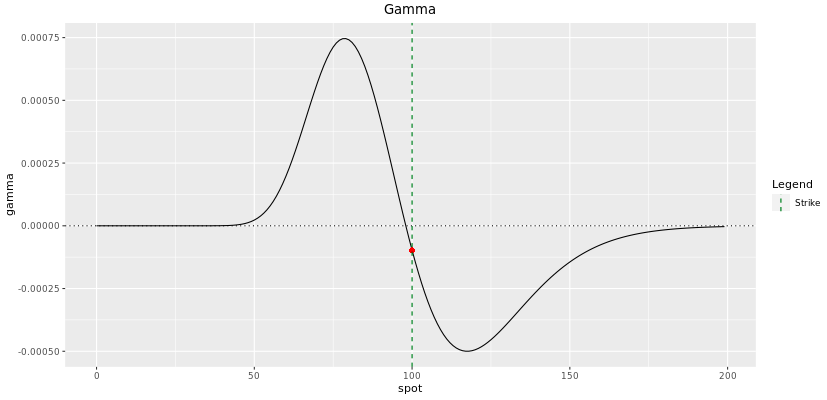##### 7.3.1.5.3 Vega

The fact that vega depends on the position of the forward price with respect to the barrier is very intuitive.

The holder of a digital call will be long volatility if the forward price is lower than the barrier level since a higher volatility will increase the probability of the spot finishing above the barrier at maturity. When the forward is lower than the barrier, you can think of the digital call as being out-of-the money. Volatility will increase the probability of the option going from OTM to ITM.

Inversely, the holder of a digital call will be short volatility if the forward price is greater than the barrier level since a higher volatility will decrease the probability of the spot finishing above the barrier at maturity. When the forward is greater than the barrier, you can think of the digital call as being in-the money. Volatility will increase the probability of the option going from ITM to OTM.##### 7.3.1.5.4 Theta

The shape of Theta plot looks completely opposite to the shape of Vega plot. This is because time to maturity has a similar effect to a digital option price as volatility. The effect is not exactly the same as time has always a second effect that comes from the discounting impact, altough this last effect is generally less important.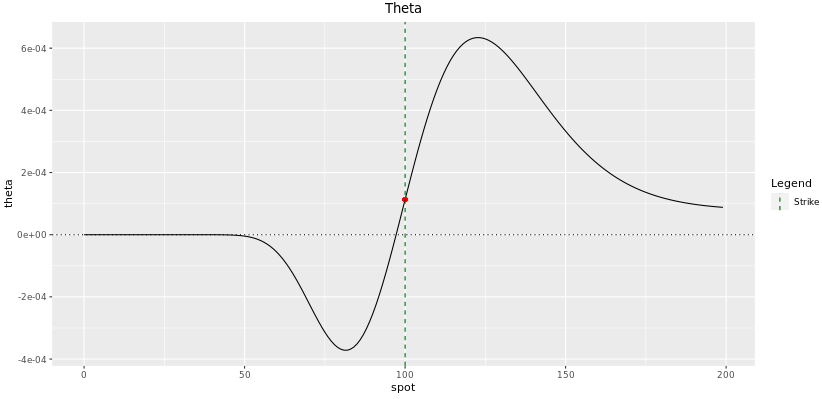Fig: 7.8 : Theta of a 1-year Digital Call at initiation

##### 7.3.1.5.5 Rho

When we spoke about Rho in section 5.6.1, we said that the effect of interest rate on an option's price came from two effects: the cost of delta-hedging and the discounting.

It is therefore not very surprising to see similarities between the Delta profile and the Rho profile. Note that the discounting effect is clearly apparent in the right-side of the curve where the option is completely in-the-money and there is no delta left. On that side of the curve, Rho is negative because an increase in interest rates inscreases the discount factor and therefore decreases the present value of the digital call. How much the Rho is negative will then mainly depend on the time to maturity.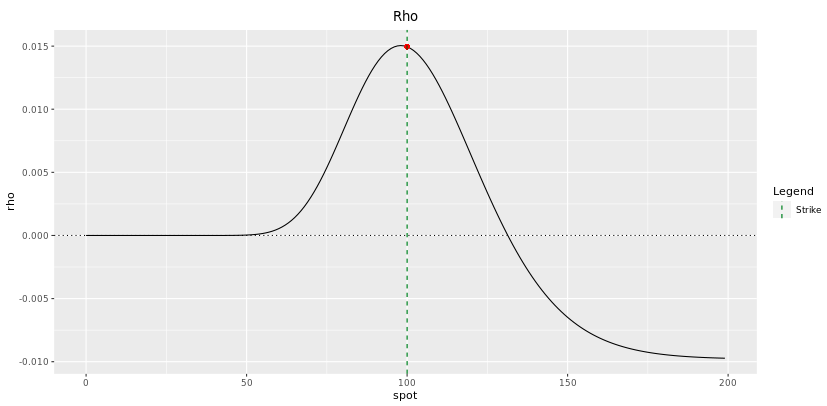Fig: 7.9 : Rho of a 1-year Digital Call at initiation

##### 7.3.1.5.6 Skew

Since a trader hedges a digital option using an option spread, the skew risk is a critical consideration.

Let us assume that we just sold a digital call, we will hedge it by buying a call spread. Taking a long position in a call spread means buying a call at a lower strike and selling a call at an upper strike. The skew makes the lower strike implied volatility more expensive than the upper strike implied volatility. Since the skew makes the hedge more expensive, it makes the structure itself more expensive. Remember what we said in section 4.5, an option price is nothing else but the cost of the hedge!

Therefore the skew makes the price of digitals more expensive. - A long position in a digital call is long the skew. - A short position in a digital call is short the skew.

Since digital options are sensitive to skew, you must use a model that knows about skew. When pricing European digitals, then your calibration should focus on getting the skew at maturity correct. When pricing American digitals with path-dependency, you will need to use some smooth surface calibration to capture the effect of surface through time. In other words, when dealing with these path-dependent american digitals, you are not only sensitive to the volatility at maturity but to many volatilities before maturity. Your volatility hedge will then consist of several European options with different maturities. We speak about vega buckets. The book of Adil Reghai is particularly good to grasp the concept of vega buckets and vega KT.

### 7.3.2 American Digitals

For American digitals, the trigger condition can be activated at anytime before maturity.

There exists a fantastic approximate link between European digital options and American digital options.

I felt quite stupid while learning about it as it is actually quite intuitive :). Back in 2015, I used to try estimating the price of every exotic option before pricing them. I was trying to develop as much as possible my intuition in terms of pricing and sensitivities in every market scenario. I quickly realised that the price of American digitals were always approximately twice the price of European digitals (with the same characteristics obviously!). The price of European digitals being quite easy to estimate, the approximations for American digitals were not too bad. One day, I decided to stay a bit later on the floor and start plotting Monte Carlo simulations to compare the price sensitivity of a barrier option to the barrier level with respect to the underlying's forward. Doing so, I realised why the above relationship between American and European digitals were so consistent. This is simply the consequence of a well-known principle followed by brownian motion: the reflection principle.

#### 7.3.2.1 The Reflection Principle

In the theory of probability for stochastic processes, the reflection principle for a Wiener process states that if the path of a Wiener process W(t) reaches a value W(s) = a at time t = s, then the subsequent path after time s has the same distribution as the reflection of the subsequent path about the value a In other words, if W(s) = a then W(t) is just as likely to be above the level a as to be below the level a for s < t.

By assuming in our models that the log-returns of the underlying are normally distributed with zero log-drift (with mean zero), the normal distribution introduces the symmetry of the reflection principle.

Gatheral expresses it nicely in his lecture on Barrier options. In Fig 6.5 below, the dashed path has the same probability of being realized as the original solid path. We deduce that the probability of hitting the barrier B is twice the probability of ending up below the barrier at expiration. Putting this another way, the value of an American digital option is twice the value of a European digital option. Note that this relationship won't be exactly respected when the log-drift is not zero (understand when the forward level is different from the spot level).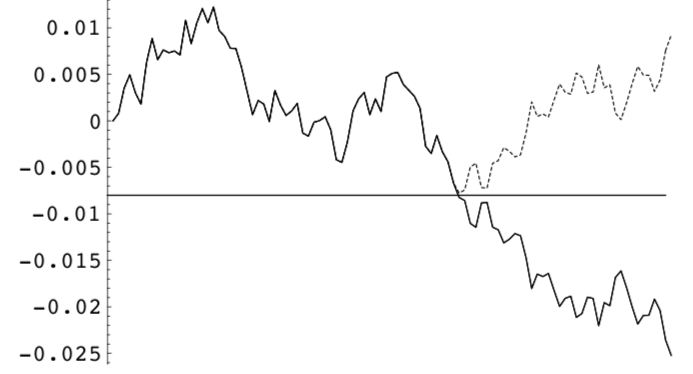#### 7.3.2.2 No-Touch Options

No-touch digital options pays a coupon if the barrier has never been touched during the option life.

It seems clear that the event of never touching the barrier is complementary to the event of ever touching it. Therefore, the probability of never touching the barrier is nothing else but 1 minus the probability of ever touching the barrier. From this parity, we can easily deduce the price of a no-touch digital option knowing the price of the american digital option and vice versa.